### 13.2.1.2 The vector case

The transformation to the phase space can be extended to differential equations in which there are time derivatives in more than one variable. Suppose that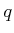represents a configuration, expressed using a coordinate neighborhood on a smooth-dimensional manifold. Second-order constraints of the form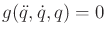or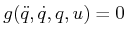can be expressed as first-order constraints in a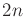-dimensional state space. Letdenote the-dimensional phase vector. By extending the method that was applied to the scalar case,is defined as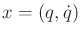. For each integersuch that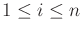,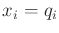. For eachsuch that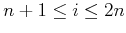,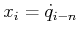. These substitutions can be made directly into an implicit constraint to reduce the order to one.

Suppose that a set ofdifferential equations is expressed in parametric form as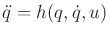. In the phase space, there aredifferential equations. The firstcorrespond to the phase space definition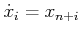, for eachsuch that. These hold because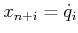and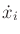is the time derivative of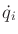for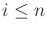. The remainingcomponents of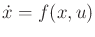follow directly from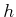by substituting the firstcomponents ofin the place ofand the remainingin the place of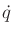in the expression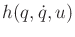. The result can be denoted as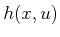(obtained directly from). This yields the finalequations as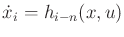, for eachsuch that. Theseequations define a phase (or state) transition equation of the form. Now it is clear that constraints on acceleration can be manipulated into velocity constraints on the phase space. This enables the tangent space concepts from Section 8.3 to express constraints that involve acceleration. Furthermore, the state spaceis the tangent bundle (defined in (8.9) for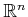and later in (15.67) for any smooth manifold) ofbecauseandtogether indicate a tangent space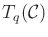and a particular tangent vector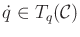.

Steven M LaValle 2020-08-14